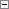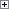Gets the day of the week, week number, or year of a Date Data Type.

Number := DATE2DWY(Date, What)

Parameters

Date

Type: Date

The input date.

What

Type: Integer

Specifies what the function returns. The valid options are 1, 2, and 3.

The value 1 corresponds to day of the week (1-7, Monday = 1).

The value 2 corresponds to week number (1-53).

The value 3 corresponds to year.Property Value/Return Value

Type: Integer

The resulting day of the week, week number, or year.Remarks

If the input date to the DATE2DWY function is in a week which spans two years, then the DATE2DWY function computes the output year as the year that has more days of the given week. For example, if the input date is 010114, then the date is in a week that starts on Monday, December 29, 2013, and ends Sunday, January 4, 2014. This week has three days in 2008 and four days in 2014. Therefore, the output year is 2014.Example

This example shows a special case that occurs when you use the DATE2DWY function in a week which spans two years. This code example requires that you create the following variables and text constants in the C/AL Globals window.

Variable name DataType

InputDate

Date

DayOfWeek

Integer

WeekNumber

Integer

Year

Integer

Text constant name ENU value

Text000

The date %1 corresponds to:\

Text001

The day of the week: %2\

Text002

The week number: %3\

Text003

The year: %4Copy Code
InputDate := 010114D;
DayOfWeek := DATE2DWY(InputDate, 1);
WeekNumber := DATE2DWY(InputDate, 2);
Year := DATE2DWY(InputDate, 3);
MESSAGE(Text000 + Text001 + Text002 + Text003, InputDate, DayOfWeek, WeekNumber, Year);

The message window displays the following:

The date 01/01/14 corresponds to:

The day of the week: 4

The week number: 1

The year: 2014

This example shows that the date 01/01/14 is regarded as day number 4 (Thursday) in week number 1 in the year 2014.See Also

Concepts

Date and Time Functions